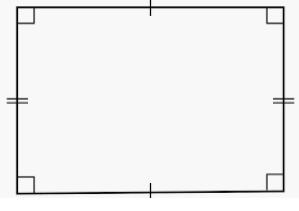# What Is The Length Of Rectangle

What is the length of the Rectangle?

A rectangle is a quadrilateral, a closed figure made of 4 line segments.

There are two pairs of opposite parallel equal sides and four right angles

There are two pairs of adjacent unequal sides in a rectangle.

The longer of the adjacent sides is called the length of the rectangle.

The shorter of the adjacent sides is called the breadth of the rectangle.Length of rectangle = L; Breadth of rectangle  = B

Perimeter of rectangle = 2(L + B); Area of rectangle = L X B